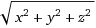I just published a suite of Vector tools on Nukepedia (Download and Quick description here).

In this post I will to run the more nerdy of you through the math involved, in part 2 I will include mini-tutorials of example usage of these tools.

### The Tools Math

Basic understanding of vectors is necessary to follow the math involved, review it here: mathsisfun.com

Luma_to_Normals: Converts an image to normals (based on Luma). This one was one I really cared about for a long time. Back in 2014 I got really close to achieve good results, but I wasn’t able to obtain the Blue channel of a normal map properly. While there are tools on Nukepedia already that attempt to achieve this, I wasn’t entirely convinced by them. I didn’t want to publish a half-baked tool, so I got back in there a few days ago and I am pretty satisfied with the result.

After some research, it seemed that the first step of converting luma to normals would be to apply some sort of Filter. I decided to use a Sobel filter. https://en.wikipedia.org/wiki/Sobel_operator
In nuke, that meant adding 2 matrix nodes, one for an horizontal filter and one vertical. I would then shuffle these into R and G.The image I will use to test the algorithm.Result of the Sobel operations. The image contains negative values that are not visible on this screenshot.

That was already sufficient for running in an iDistort for example to fake a refraction or heat haze, etc.. That’s what I had been using for a long time. However, for a complete tool, I needed to get 3D vectors, not 2D.
As normal maps are vectors representing a direction, the magnitude of their vectors is normalized so that each magnitude is 1.
The math to calculate a vector magnitude is.
Since I knew X and Y, and wanted a final magnitude of 1, I had to write the expression, which solves Z like this.
I wrote the expression in Nuke. Now the problem is that if the magnitude of the 2D vector was already longer than 1, the expression would error and add a lot of “nan”. So I added an expression in order to return 0 in blue if the 2D vector was already 1 or longer.
By adding a grade node before the Sobel operation, it’s possible to control the overall magnitude of the 2D vectors, allowing to reduce the errors happening when calculating the 3D vector. I also added some extra controls, packed it in a group, and the tool was done.The resulting normals (displayed in a 0-1 range)

UV_Map_Generator: This one was pretty straight forward. UV maps are ramps that go from 0 to 1 horizontally in R, and vertically in G.
The one little trick was that Nuke calculates the X and Y position of pixels from the bottom left corner of the pixel, while STMaps and other UV based tools calculate it from the center of the Pixel. I used to generate them with the expression r = x/width, g=y/height. To be correct I had to add 0.5 to x and y to center the value to the pixel. So the final expression is r = (x+.5)/width, g=(y+.5)/height.

UV_to_Vectors: By subtracting a distorted UV map and an original UV map, we obtain some sort of faint vectors corresponding to the distortion, however, to truly get the right magnitude, it’s necessary to multiply r by the width and g by the height.
So for the expression, onto a distorted map (in r and g), I need to re-generate an original UV to subtract to the distorted UV:
r = (r-((x+.5)/width))*width
g = (g-((y+.5)/height))*height
Which can be simplified to:
r = -x+r*width-0.5
g = -y+g*height-0.5
(I’m using http://www.wolframalpha.com to simplify my expressions, because I’m lazy)

Vectors_to_UV: Basically the opposite of above:
r = (r+x+0.5)/width
g = (g+y+0.5)/height

Vectors_Direction: I wanted a way to rotate 2D vectors. No need to re-invent the wheel, I googled the formula, and wrote it into a Nuke expression. Sadly I can’t cite my source anymore since I found it years a go and can’t recall where I found it from. The thing to be aware is that the formula expected angles in radians, so the first thing I did is define a variable, then use it into the expression.